Next: 4.2.2 Electron-Phonon Coupling Up: 4.2 Formalism Previous: 4.2 Formalism

## 4.2.1 Model

The Holstein Hamiltonian in real space is given by(4.1)

where() is the electron (phonon) annihilation operator at site. The first term describes the electron kinetic energy. The second term describes a set of independent oscillators with frequency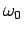at every site. The electron-phonon coupling in the Holstein model is local and is described by the last term of Eq. 4.1 where the electron densitycouples with the lattice displacementwith strength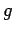.

Our breathing Hamiltonian is a one-dimensional version of the model which describes the coupling of Zhang-Rice (ZR) singlets (29) with the Cu-O bond-stretching vibrations in high Tsuperconductors (22,23). In cuprates a ZR singlet is a bound state between a hole on the Cu and a hole on the four neighboring O atoms. It's energy is stabilized by the Cu-O hybridization term and therefore is influenced by the Cu-O distance. In our one dimensional model we consider a set of independent, in-between sites oscillators (the analogue of the O atoms) which modulate the charge carrier's (the analogue of the ZR singlet) on-site energy. Therefore we define the B Hamiltonian as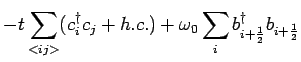(4.2)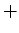Both the H and the B model can be written in the momentum representation as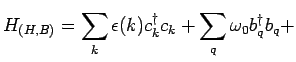(4.3)where(4.4)

is the H electron-phonon coupling and(4.5)

is the B electron-phonon coupling. Notice that in the momentum representation the H coupling is a constant and the B coupling is an increasing function of the phonon momentum for small momenta.

In Eq. 4.1 and Eq. 4.2 the free electron part of the Hamiltonian was introduced as a tight-binding hopping. However in order to study the influence of electron dispersion on the polaron properties in this chapter we also employ calculations which consider different forms of electron dispersion.Next: 4.2.2 Electron-Phonon Coupling Up: 4.2 Formalism Previous: 4.2 Formalism
© Cyrill Slezak